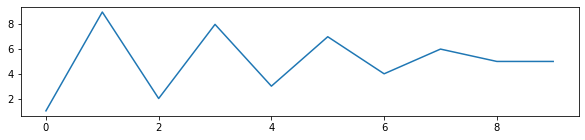👋 Hello! I'm Alphonsio the robot. Ask me a question, I'll try to answer.

# In Python, how do you change the size of figures drawn with Matplotlib?

The simplest way to change the size of an existing Matplotlib figure is to use the method ﻿set_size_inches():

import matplotlib.pyplot as plt
plt.plot ([1,9,2,8,3,7,4,6,5,5])

# Set figure size in inches
fig = plt.gcf()
fig.set_size_inches(20, 4)

The above code should display something like:More